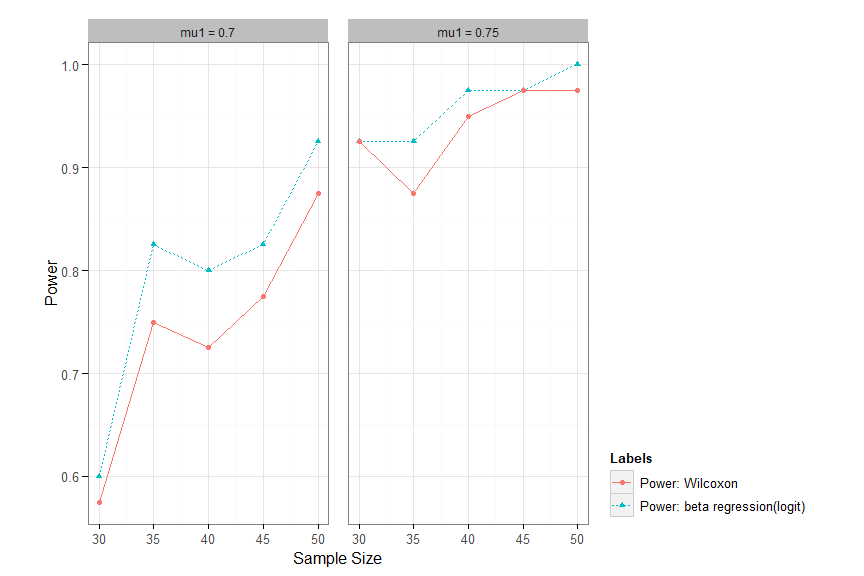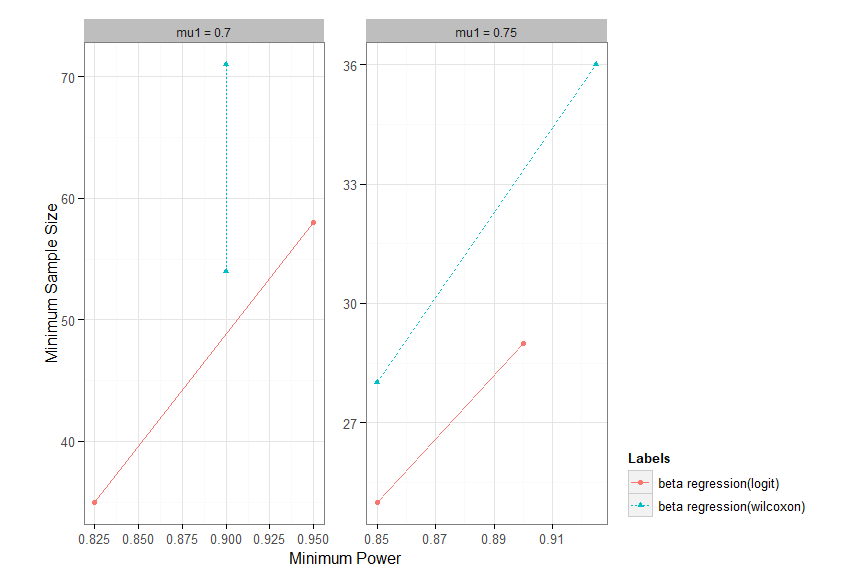# Calculating Power and Sample Size with BetaPASS

## Important Notes

An updated version is available on CRAN as PASSED.

## Introduction

Assume the response variable $$Y$$ is a proportion or otherwise constrained between 0 and 1 which can be modeled with a beta dbn. This program will help find the power for a given sample size or the sample size given power, when testing the null hypothesis that the means for the control and treatment groups are equal against a two-sided alternative. The user must supply the mean and standard deviation for the control group ($$\mu_0$$ and $$sd_0$$) as well as the mean for the treatment group under the alternative, namely $$\mu_1$$.

If $$Y \sim beta(a,b)$$, then $$\mu=\frac{a}{a+b}$$ and the variance of $$Y$$ can be expressed in terms of $$\mu$$ using the parameter $$\phi$$ as $$Var(Y)=\frac{\mu {(1-\mu)}}{1+\phi}$$. The value of $$\phi$$ is found from $$\mu_0$$ and $$sd_0$$. That value is then used to find the variance under the alternative. Given $$\mu$$ and $$\phi$$ the parameters $$a$$ and $$b$$ can be found from $$a = \mu \phi$$ and $$b=(1-\mu) \phi$$. The values of $$a$$ and $$b$$ are then used to generate random beta variables for the simulation.

## Calculating Power

To illustrate this we use an example. A client proposed a study with an intervention designed to increase ‘adherence’ to protocols designed to minimize effects of lymphedema (LE). Each patient has her own set of activities to be done on a regular basis. The adherence score is based on a weekly diary where the subject notes which activities were done each day. The client has not used the diary before and has no pilot information. Nevertheless she wants to know what sample size (for the treatment group and the control group) is needed to have ‘good power’. The client did find a similar study (using a different diary) that gave the following information:

Collectively, BCRL self-care adherence among the 128 women prescribed with one or more lymphedema self-care modality was as follows:

16 (13$$\%$$) reported a mean of less than 25$$\%$$ of adherence,

36 (28$$\%$$) reported a mean of 25$$\%$$-49$$\%$$ of adherence,

40 (31$$\%$$) reported a mean of 50$$\%$$-74$$\%$$ of adherence,

and 36 (28$$\%$$) reported a mean of 75$$\%$$-100$$\%$$ of adherence.

Using the mid-points of the intervals we have the following:

Range Mid-pt Prob
0-.25 .125 0.13
.25-.50 .375 0.28
.50-.75 .625 0.31
.75-1.0 .875 0.28

Treating this as a probability distribution, the mean and variance are 0.56 and 0.0625. A beta distribution with a=1.56 and b=1.22 (or $$\mu$$=0.56 and $$\phi$$=2.78) would have the same mean and variance.

Range Mid-pt Prob Beta model
0-.25 .125 0.13 0.144
.25-.50 .375 0.28 0.263
.50-.75 .625 0.31 0.312
.75-1.0 .875 0.28 0.281

The client thought that a difference of 0.56 in the control group and 0.70 or 0.75 in the treatment group would be clinically important. If the mean and variance of the beta distribution under Ho are 0.56 and 0.0625, what sample sizes would give good power under Ha: $$\mu$$=0.70 or 0.75?

This problem can be addressed parametrically or nonparametrically. Non-parametric method uses Wilcoxon Rank sum test. A parametric approach is to assume the underlying distribution is beta. Then generate beta data under the null hypothesis and under the alternative and run simulations to estimate the probability of rejecting the null hypothesis based on GLM method.

You can vary the sample size and/or the alternative.

In this case, you can run following codes (if necessary, first install the BetaPASS and prerequisite library):

if (!require(BetaPASS)){
devtools::install_github("CastleLi/draft/BetaPASS")
Needed_packages <- c("Rcpp","betareg","ggplot")
install.packages(Needed_packages)
}

Next load the BetaPASS, and then use betapower function:

library(BetaPASS)
Power.mat <- betapower(mu0 = 0.56, sd0 = 0.255,
mu1.start = .70, mu1.end = .75, mu1.by = .05,
ss.start = 30, ss.end = 50, ss.by = 5,
trials = 40, seed = 1,
link.type = c("logit"))

The output will give the estimated power for each sample size and alternative mean combination, for both parametrical and non-parametrical approach.

beta regression(logit) Wilcoxon sample size mu1
0.600 0.575 30 0.70
0.925 0.925 30 0.75
0.825 0.750 35 0.70
0.925 0.875 35 0.75
0.800 0.725 40 0.70
0.975 0.950 40 0.75
0.825 0.775 45 0.70
0.975 0.975 45 0.75
0.925 0.875 50 0.70
1.000 0.975 50 0.75

You can generate the plots comparing the power using the Wilcoxon Rank Sum test and GLM method with following codes:

plot(Power.mat, link.type = "logit", by = "mu1")It appears that the parametric test does better(a savings of about 10% in sample size).

## Calculating Sample Size

Also you can calculate the minimum sample size directly with given power and alternative using following codes:

samplesize(mu0=0.56, sd0=0.255, mu1.start = 0.75, power.start = 0.8, trials = 40,
#>  "An updated version is available on CRAN as PASSED.\n Please check CRAN for more details."
#>       Two beta-distributed samples sample size calculation
#>
#>               mu0 =  0.56
#>               sd0 =  0.255
#>         sig.level =  0.05
#>  number of trials =  40
#>
#>       Minimum sample size(corresponding power)
#>      beta regression(logit) Wilcoxon target power mu1
#> [1,] 25(0.85)               28(0.85) 0.8          0.75

If you want to compare the minimum sample sizes with different powers and alternatives, or different types of link, you can use following codes:

SS.mat <- samplesize(mu0=0.56, sd0=0.255,
mu1.start = 0.70, mu1.end = 0.75, mu1.by = 0.05,
power.start = 0.8, power.end = 0.9, power.by = 0.1,
trials = 40, link.type = c("logit","wilcoxon"))
#>  "An updated version is available on CRAN as PASSED.\n Please check CRAN for more details."

The output will give the estimated sample size for each target power and alternative mean combination.

minimum sample size: logit minimum power: logit minimum sample size: wilcoxon minimum power: wilcoxon target power mu1
35 0.825 54 0.900 0.8 0.70
25 0.850 28 0.850 0.8 0.75
58 0.950 71 0.900 0.9 0.70
29 0.900 36 0.925 0.9 0.75

You can generate the plots comparing the sample size with following codes:

plot(SS.mat, link.type = c("logit","wilcoxon"))